### Home > CALC > Chapter 5 > Lesson 5.1.3 > Problem5-25

5-25.
1. Use the graphs of f ′(x) and g′(x) below to determine the x-values of all local minimums, maximums, and points of inflection. Homework Help ✎

1.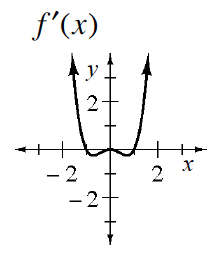2.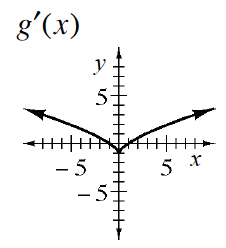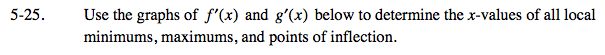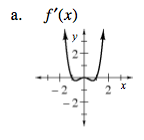f(x) will be increasing where f '(x) is positive, and vice versa.
f(x) will be concave up where f '(x) has positive slopes, and vice versa.

Local max at x = −1; f'(x) changes from positive to negative.
Inflection points at x = −0.5, 0, 0.5; f '(x) changes slope.
Local min at x = 1; f '(x) changes from negative to positive.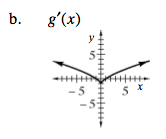See the hint in part (a).